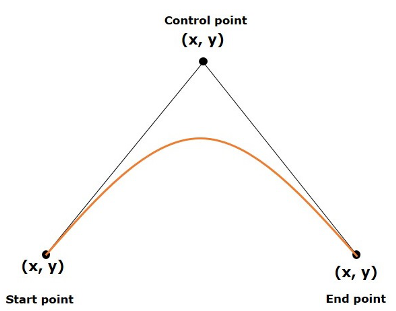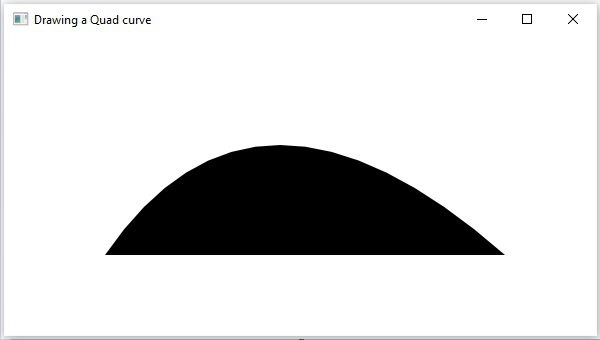# JavaFX - 2D Shapes Quad Curve

Mathematically a quadratic curve is one that is described by a quadratic function like − y = ax2 + bx + c.

In computer graphics Bezier curves are used. These are parametric curves which appear reasonably smooth at all scales. These Bezier curves are drawn based on points on an XY plane.

A quadratic curve is a Bezier parametric curve in the XY plane which is a curve of degree 2. It is drawn using three points: start point, end point and control point as shown in the following diagramIn JavaFX, a QuadCurve is represented by a class named QuadCurve. This class belongs to the package javafx.scene.shape.

By instantiating this class, you can create a QuadCurve node in JavaFX.

This class has 6 properties of the double datatype namely −

• startX − The x coordinate of the starting point of the curve.

• startY − The y coordinate of the starting point of the curve.

• controlX − The x coordinate of the control point of the curve.

• controlY − The y coordinate of the control point of the curve.

• endX − The x coordinate of the end point of the curve.

• endY − The y coordinate of the end point of the curve.

To draw a QuadCurve, you need to pass values to these properties. This can be done either by passing them to the constructor of this class, in the same order, at the time of instantiation, as follows −

```QuadCurve quadcurve = new QuadCurve(startX, startY, controlX, controlY, endX, endY);
```

Or, by using their respective setter methods as follow −

```setStartX(value);
setStartY(value);
setControlX(value);
setControlY(value);
setEndX(value);
setEndY(value);
```

To Draw a Bezier Quadrilateral Curve in JavaFX, follow the steps given below.

### Step 1: Creating a Class

Create a Java class and inherit the Application class of the package javafx.application. Then you can implement the start() method of this class as follows.

```public class ClassName extends Application {
@Override
public void start(Stage primaryStage) throws Exception {
}
}
```

### Step 2: Creating a QuadCurve

You can create a QuadCurve in JavaFX by instantiating the class named QuadCurve which belongs to a package javafx.scene.shape. You can then instantiate this class as shown in the following code block.

```//Creating an object of the class QuadCurve
```

### Step 3: Setting Properties to the QuadCurve

Specify the x, y coordinates of the three points: start point, end point and control points, of the required curve, using their respective setter methods as shown in the following code block.

```//Adding properties to the Quad Curve
```

### Step 4: Creating a Group Object

In the start() method, create a group object by instantiating the class named Group, which belongs to the package javafx.scene.

Pass the QuadCurve (node) object created in the previous step as a parameter to the constructor of the Group class, in order to add it to the group as follows −

```Group root = new Group(quadcurve);
```

### Step 5: Creating a Scene Object

Create a Scene by instantiating the class named Scene which belongs to the package javafx.scene. To this class pass the Group object (root) created in the previous step.

In addition to the root object, you can also pass two double parameters representing height and width of the screen along with the object of the Group class as follows.

```Scene scene = new Scene(group ,600, 300);
```

### Step 6: Setting the Title of the Stage

You can set the title to the stage using the setTitle() method of the Stage class. The primaryStage is a Stage object which is passed to the start method of the scene class, as a parameter.

Using the primaryStage object, set the title of the scene as Sample Application as follows.

```primaryStage.setTitle("Sample Application");
```

### Step 7: Adding Scene to the Stage

You can add a Scene object to the stage using the method setScene() of the class named Stage. Add the Scene object prepared in the previous steps using this method as follows.

```primaryStage.setScene(scene);
```

### Step 8: Displaying the Contents of the Stage

Display the contents of the scene using the method named show() of the Stage class as follows.

```primaryStage.show();
```

### Step 9: Launching the Application

Launch the JavaFX application by calling the static method launch() of the Application class from the main method as follows.

```public static void main(String args[]){
launch(args);
}
```

### Example

Following is a program which generates a quadrilateral curve using JavaFX. Save this code in a file with the name QuadCurveExample.java.

```import javafx.application.Application;
import javafx.scene.Group;
import javafx.scene.Scene;
import javafx.stage.Stage;

public class QuadCurveExample extends Application {
@Override
public void start(Stage stage) {

//Creating a Group object

//Creating a scene object
Scene scene = new Scene(root, 600, 300);

//Setting title to the Stage

stage.setScene(scene);

//Displaying the contents of the stage
stage.show();
}
public static void main(String args[]){
launch(args);
}
}
```

Compile and execute the saved java file from the command prompt using the following commands.

```javac QuadCurveExample.java